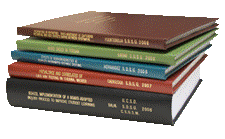Archival Master’s Theses from the University of Nebraska-Lincoln

Thesis

7-1940

Citation

Thesis (M.A.)—University of Nebraska—Lincoln, 1940. Department Mathematics.

Copyright 1940, the author. Used by permission.

Abstract

This paper treats the solution of algebraic equations by transforming the original equation into an equation whose roots are powers of the roots of the original equation.

Dandelin, Graeffe, and Lobachevsky have developed the root squaring method in which the transformed equation has Encke roots which are squares of roots of the original equation. This new equation is then transformed, and the resulting equation has Encke roots which are the squares of roots of the second equation, and the fourth power of the roots of the original equation.By repeated transformation one gets the eighth, sixteenth, thirty-second, etc., power of the original roots. If the original roots are unequal, as they are raised to higher and higher powers, they both become more and more widely separated.

The purpose of this paper is to generalize the root squaring method of Dandelin, so that equations are transformed into new equations whose roots are cubes or fourth powers of those of the preceding equation, rather than squares.To use the root cubing process, one transforms the given equation into an equation whose roots are cubes of the given roots. Repeating the process gives an equation whose roots are the ninth powers of the given roots. Continuing the process, one gets the 27th, 81st, etc., powers of the given roots.

The root cubing method will require fewer transformations than are needed in root squaring to reach the required power of the original equation.The sign of the numerical result of root squaring must be determined by substitution, by graph, or by some other method, while root cubing will give the sign as well as the numerical part of the root.For this reason, the root cubing method will be particularly emphasized in this thesis.